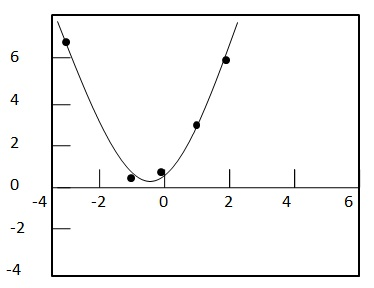# Statistics - Quadratic Regression Equation

#### Class 11th Statistics for Economics

40 Lectures 3.5 hours

#### Statistics

40 Lectures 2 hours

#### An Introduction to Wait Statistics in SQL Server

22 Lectures 1 hours

Quadratic regression is deployed to figure out an equation of the parabola which can best fit the given set of data. It is of following form:

${ y = ax^2 + bx + c \ where \ a \ne 0}$

Least square method can be used to find out the Quadratic Regression Equation. In this method, we find out the value of a, b and c so that squared vertical distance between each given point (${x_i, y_i}$) and the parabola equation (${ y = ax^2 + bx + c}$) is minimal. The matrix equation for the parabolic curve is given by:

${\begin{bmatrix} \sum {x_i}^4 & \sum {x_i}^3 & \sum {x_i}^2 \\ \sum {x_i}^3 & \sum {x_i}^2 & \sum x_i \\ \sum {x_i}^2 & \sum x_i & n \end{bmatrix} \begin{bmatrix} a \\ b \\ c \end{bmatrix} = \begin{bmatrix} \sum {x_i}^2{y_i} \\ \sum x_iy_i \\ \sum y_i \end{bmatrix} }$

## Correlation Coefficient, r

Correlation coefficient, r determines how good a quardratic equation can fit the given data. If r is close to 1 then it is good fit. r can be computed by following formula.

${ r = 1 - \frac{SSE}{SST} \ where \\[7pt] \ SSE = \sum (y_i - a{x_i}^2 - bx_i - c)^2 \\[7pt] \ SST = \sum (y_i - \bar y)^2 }$

Generally, quadratic regression calculators are used to compute the quadratic regression equation.

### Example

Problem Statement:

Compute the quadratic regression equation of following data. Check its best fitness.

 x y -3 -2 -1 0 1 2 3 7.5 3 0.5 1 3 6 14

Solution:

Compute a quadratic regression on calculator by putting the x and y values. The best fit quadratic equation for above points comes as

${ y = 1.1071x^2 + x + 0.5714 }$

To check the best fitness, plot the graph.So the value of Correlation Coefficient, r for the data is 0.99420 and is close to 1. Hence quadratic regression equation is best fit.